# Exploratory Data Analysis (1)

## Introduction

In this Chapter, we will begin to explore the EDA functionality in GeoDa, in particular the methods that deal with one or two variables. We leave the treatment of multiple variables for the next Chapter. We also illustrate the powerful linking and brushing capability that is central to the architecture of the program. We start with the description and visualization of a single variable, and then move on to the bivariate scatter plot.

We will use a data set with demographic and socio-economic information for 55 New York City sub-boroughs. The data are from the Furman Institute at NYU. This data set is part of the GeoDa Sample Data and can be loaded directly into the program.

### Objectives

• Computing descriptive statistics and creating visualizations of the distribution of a single variable (histogram, box plot)

• Interpreting a scatter plot and scatter plot smoothing (LOWESS)

• Carrying out scatter plot brushing and linking

• Assessing spatial heterogeneity through the Chow test

#### GeoDa functions covered

• Linking and brushing graphs and maps
• Variable settings dialog
• Explore > Histogram
• Choose Intervals option
• View > Display Statistics option
• Save Image as option
• Explore > Box Plot
• Hinge option
• Explore > Scatter Plot
• View > Display Precision option
• Data option
• Smoother option
• LOWESS parameters setting
• Regimes Regression option and Chow test

### Getting started

We open GeoDa and select (double click on the thumbnail map icon) the data set NYC Data from the list contained under the Sample Data tab, as shown in Figure 1.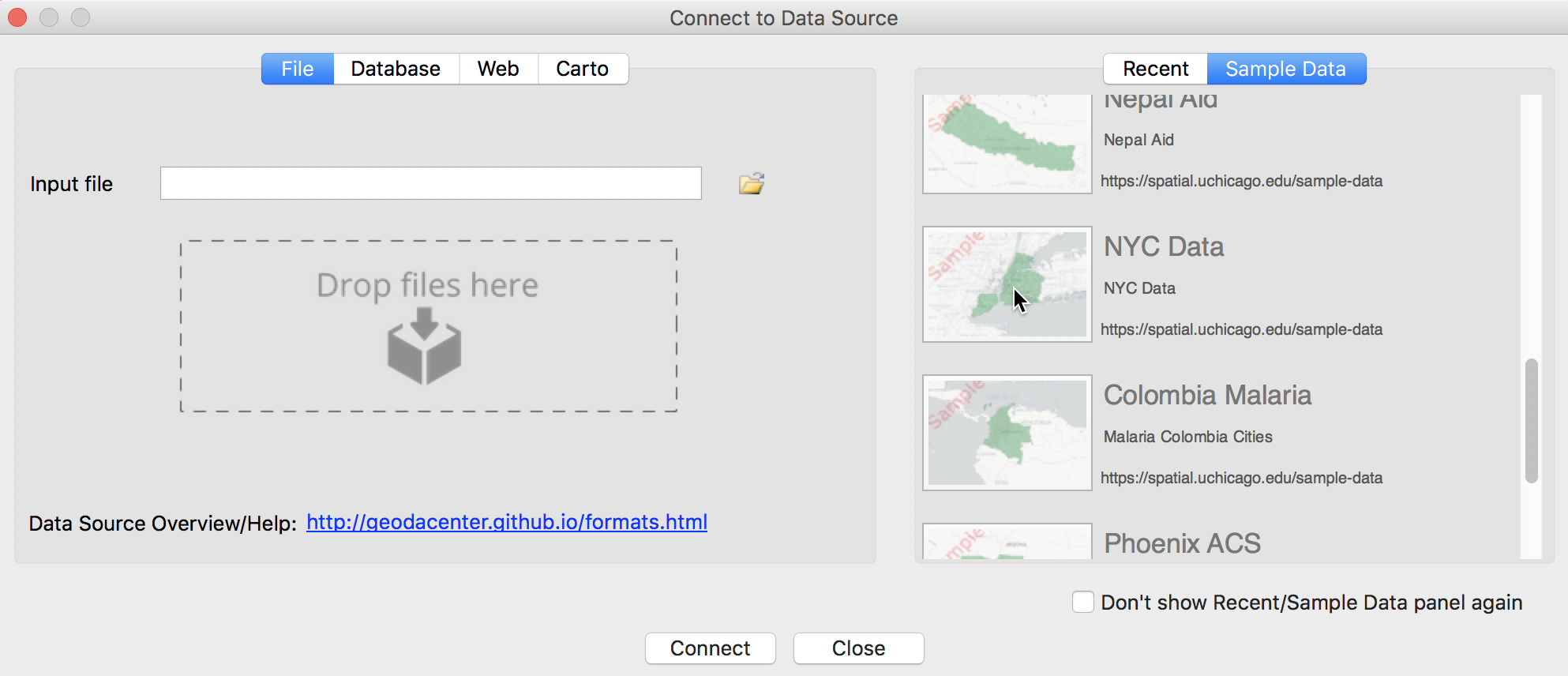Figure 1: NYC Data sample data set

This opens up a green themeless map of the 55 New York City sub-boroughs (Figure 2).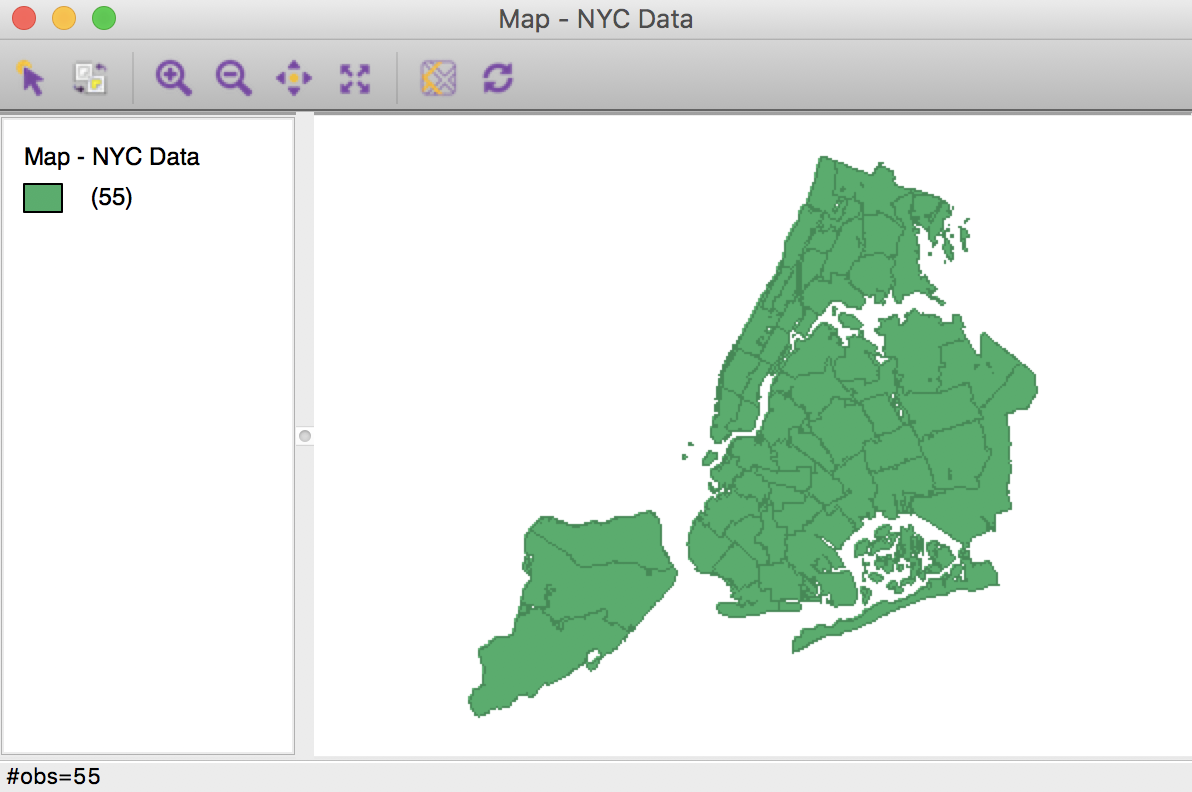Figure 2: NYC sub-boroughs

We will focus our attention on the functionality of the Explore menu, listed in Figure 3.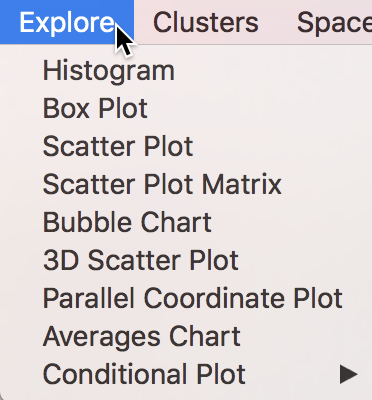The counterpart to the menu items are the collection of eight icons on the main toolbar, shown in Figure 4. The icon for the Averages Chart is separate. We postpone the treatment of this tool until a later chapter.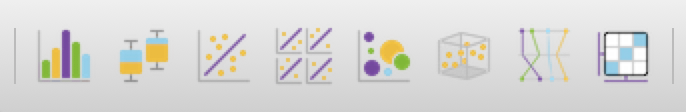Figure 4: Explore toolbar icons

The first two icons on the left pertain to univariate analyses, respectively the Histogram and Box Plot. The Scatter Plot extends this to bivariate association. The other icons pertain to the analysis of multiple variables, which we leave to the next Chapter to consider.

## Analyzing the Distribution of a Single Variable

### Histogram

We begin our analysis with the simple description of the distribution of a single variable. Arguably the most familiar statistical graphic is the histogram, which is a discrete representation of the density function of a variable. In essence, the range of the variable (the difference between maximum and minimum) is divided into a number of equal intervals (or bins), and the number of observations that fall within each bin is depicted in a bar graph.

The histogram functionality is started by selecting Explore > Histogram from the menu, or by clicking on the Histogram toolbar icon, the left-most icon in the set in Figure 5.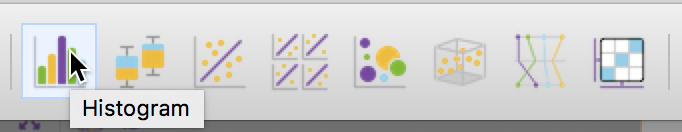Figure 5: Histogram toolbar icon

This brings up the Variable Settings dialog, which lists all the numeric variables in the data set (string variables cannot be analyzed). Scroll down the list as in Figure 6 until you can select kids2009, the percentage of households with kids under age 18 in 2009.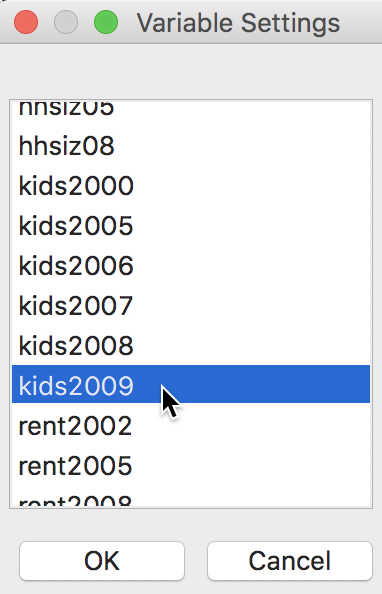Figure 6: Histogram variable selection

After clicking OK, the default histogram appears, showing the distribution of the 55 observations over seven bins, as in Figure 7. The distribution tends to be skewed to the left, with a tail on the low end and most taller bins on the high end, suggesting more areas with a higher percentage of kids under 18.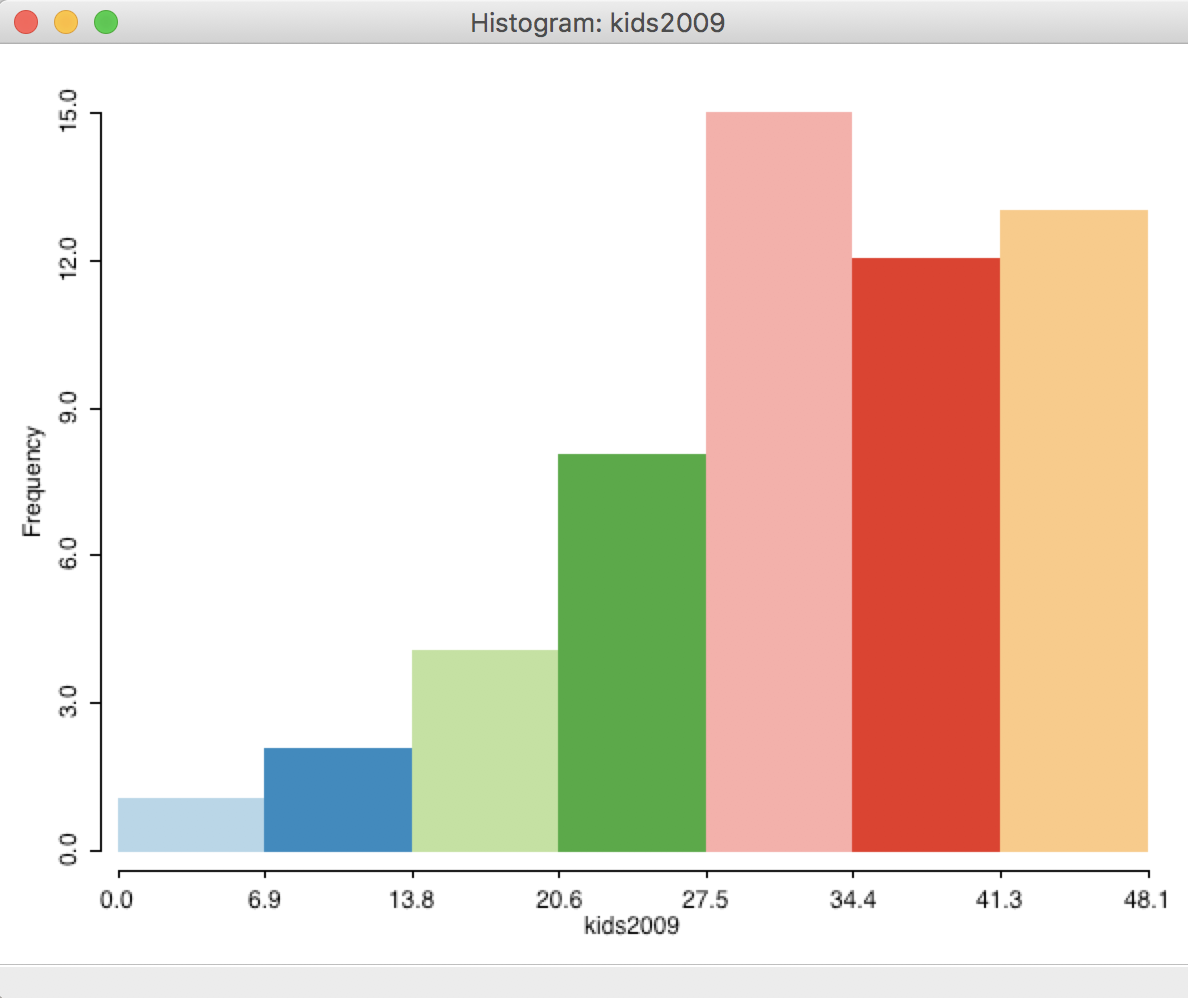Figure 7: Default histogram

There are two important options for the histogram. One is to set the number of bins for the default equal interval setting, the other is to customize the values of the cut-off points completely. We will postpone a consideration of the latter until the discussion of choropleth maps (in essence, a map version of a histogram).

The histogram options shown in Figure 8 are brought up in the usual fashion, by right clicking on the graph.Figure 8: Choose intervals histogram option

#### Selecting the number of histogram bins

After selecting the Choose Intervals option, a dialog appears that lets you set the number of bins explicitly. The default is 7, but in our example, we change this to 5, as in Figure 9.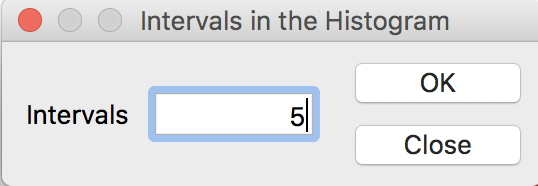Figure 9: Histogram intervals set to 5

The resulting histogram now has five bars, as in Figure 10.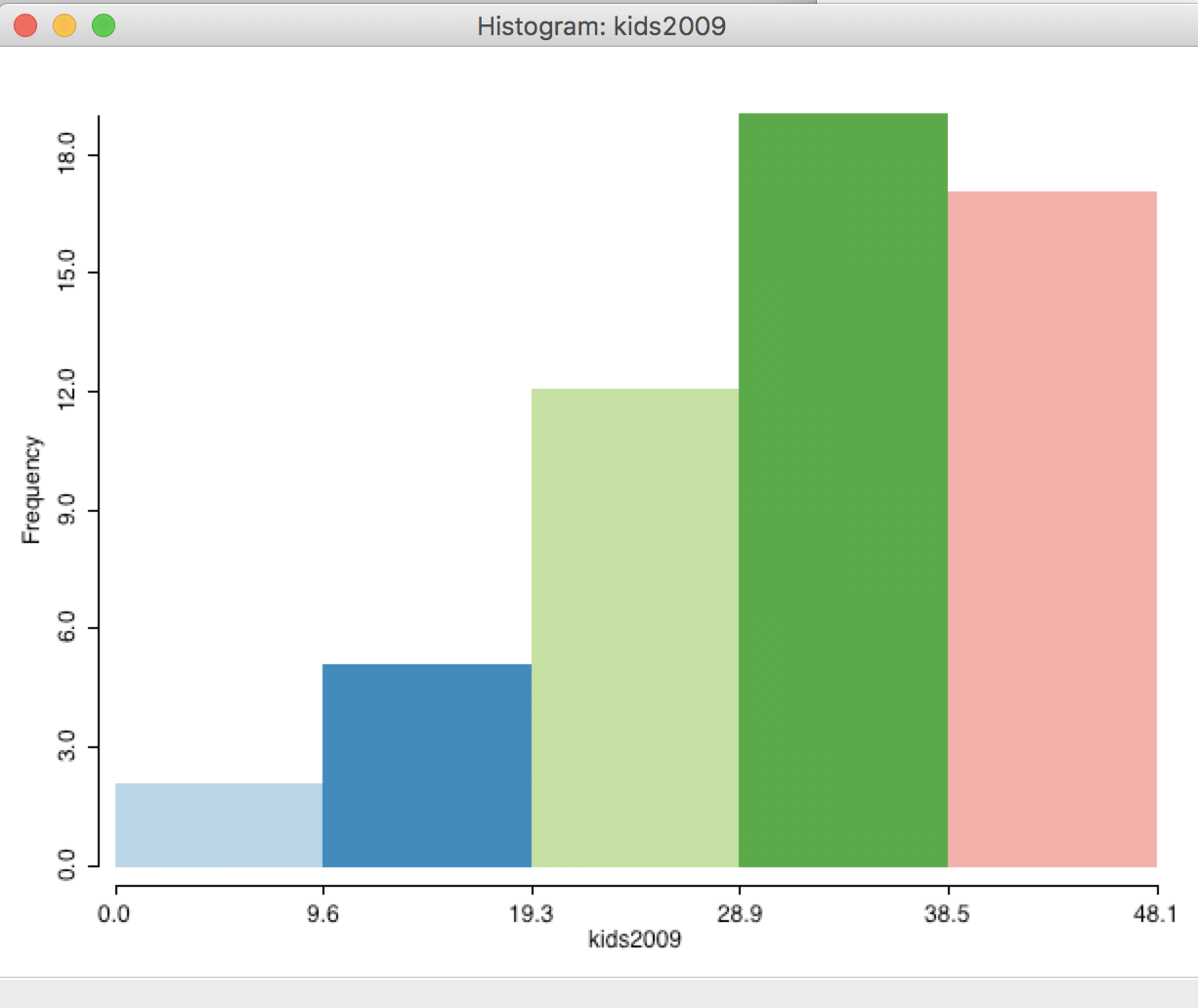Figure 10: Histogram with 5 intervals

Note how the wider bins for the histogram somewhat smooth the shape of the distribution.

#### Display histogram statistics

A second important option for the histogram (and any other statistical graph) is to display descriptive statistics in the graph. This is accomplished by selecting View > Display Statistics in the option menu shown in Figure 11.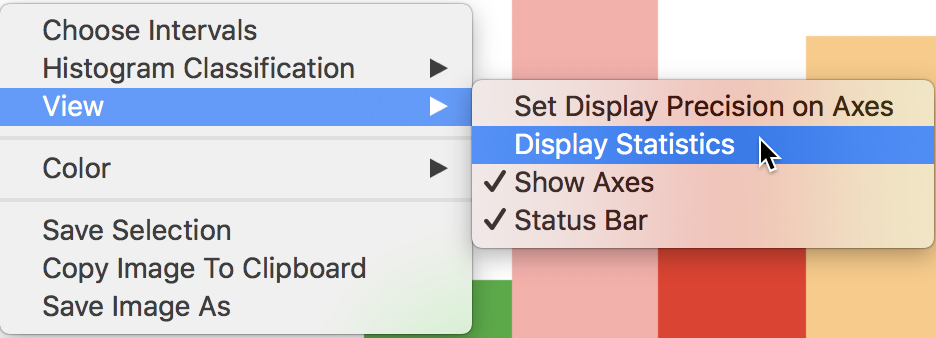Figure 11: Display statistics option

The Display Statistics option adds a number of descriptors below the graph. The summary statistics are given at the bottom, illustrated in Figure 12. We see that the 55 observations have a minimum value of 0, a maximum of 48.1, median of 33.5, mean of 32.1 and a standard deviation of 10.4. We take the minimum value as is, even though a percentage of zero may seem suspicious. In addition, for the histogram, descriptive statistics are provided for each interval, showing the range for the interval, the number of observations as a count and as a percentage of the total number of observations, and the number of standard deviations away from the mean for the center of the bin. This allows us to identify potential outliers, e.g., as defined by those observations more than two standard deviations from the mean. In our example, the lowest category would satisfy this criterion.

The summary characteristics for a given bin also appear in the status bar when the cursor is moved over the corresponding bar. This works whether the descriptive statistics option is on or not. In our example in Figure 12, the cursor is over the central bar.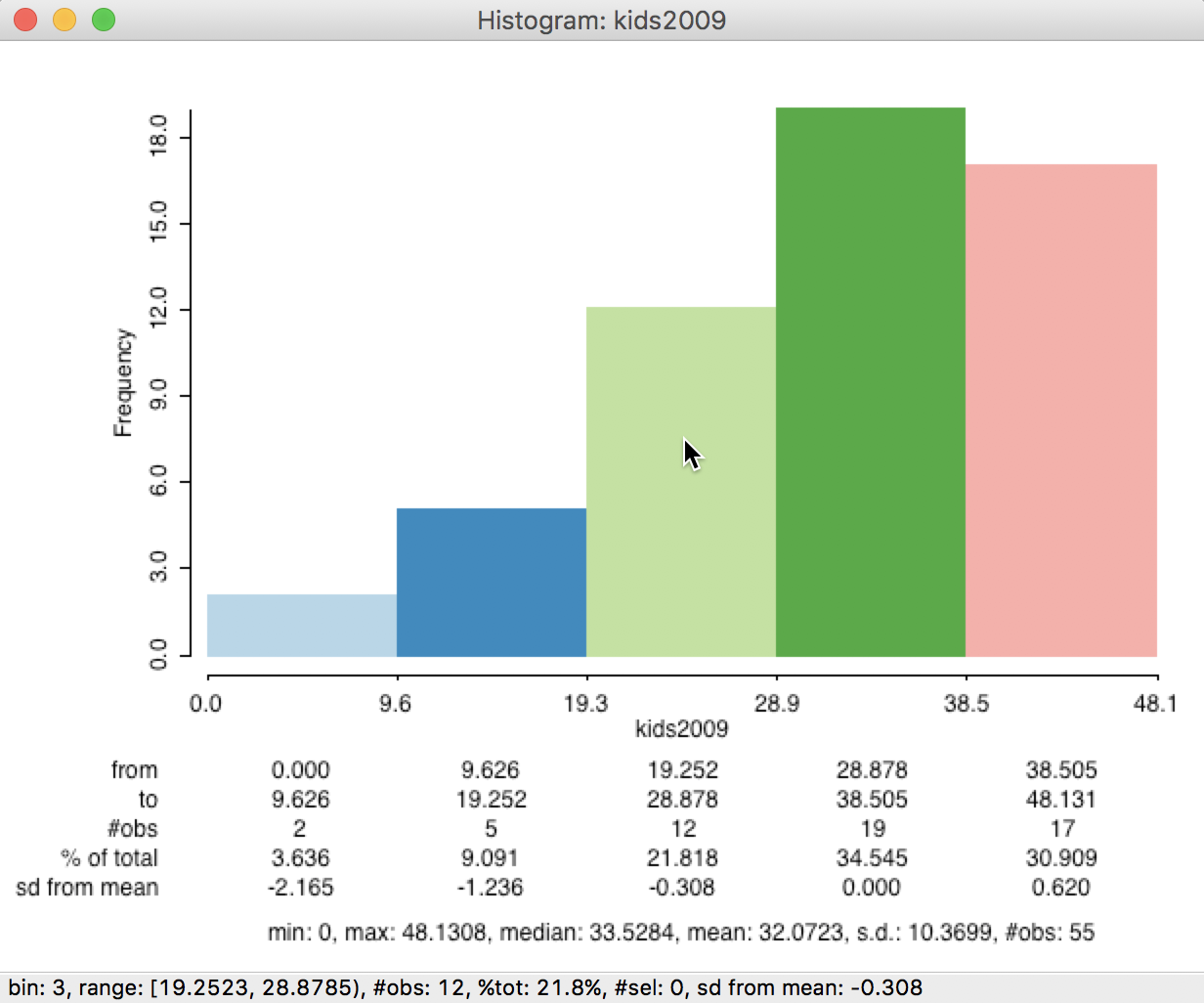Figure 12: Histogram with descriptive statistics

Other options available in the Histogram are adjustments to various color settings (Color), saving the selection (see below), Copy the Image to Clipboard and saving the graph as an image file (see below).

#### Linking a histogram and a map

To illustrate the concept of linked graphs and maps, we first set the number of intervals for the histogram back to 7 (Choose Intervals > 7). Then we select the three left-most bars in the histogram (click and shift-click to expand the selection). The highlighted bars keep their color, whereas the non-selected ones become transparent, as in the right-hand graph in Figure 13. This is the standard approach to visualize a selection in a graph in GeoDa.2

Immediately upon selection of the bars in the graph, the corresponding observations in the map are also highlighted, as in the left-hand graph in Figure 13. In our current example, the map is a simple themeless map (all areal units are green), but in more realistic applications, the map can be any type of choropleth map, for the same variable or for a different variable. The latter can be very useful in the exploration of categorical overlap between variables.

In our example, the histogram bars at the low end of the distributions (i.e., with a low percentage of households with kids) correspond to sub-boroughs primarily located in Manhattan, which should not come as a surprise.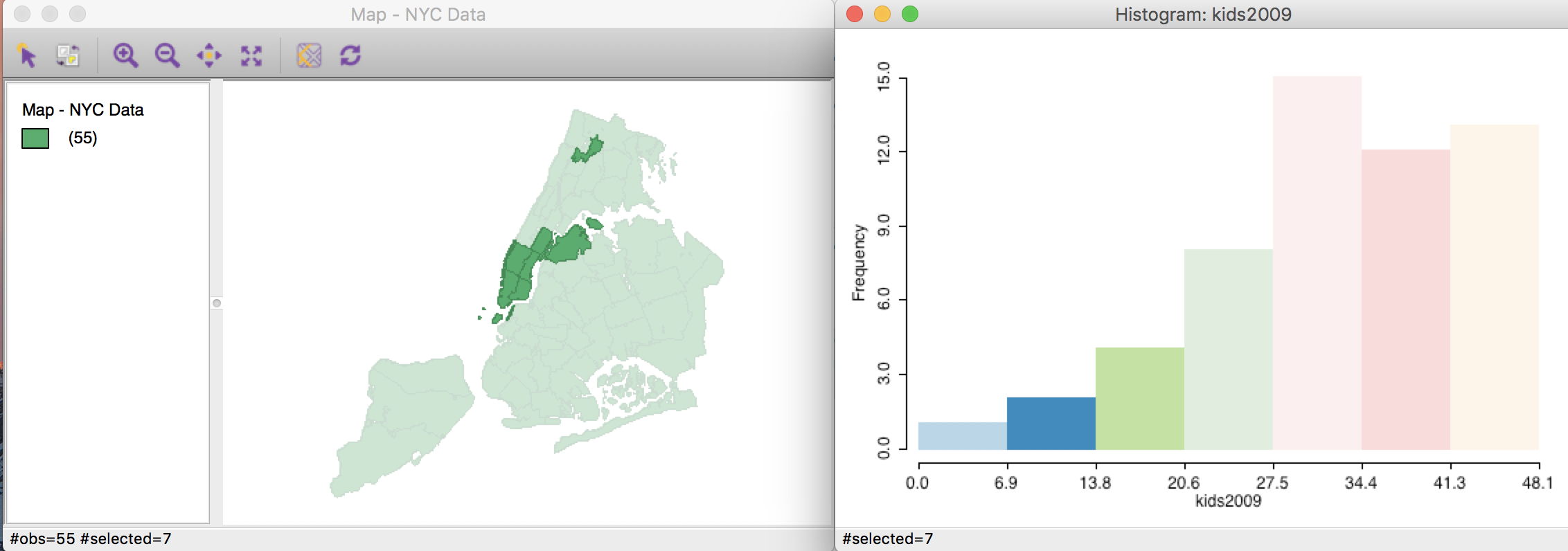Figure 13: Linking a histogram and a map

The reverse linking works as well. For example, using a rectangular selection tool on the themeless map, we can select sub-boroughs in Manhattan and adjoining Brooklyn, as in the map in Figure 14. The linked histogram (right-hand graph in Figure 14) will show the attribute distribution for the selected spatial units as highlighted fractions of the bars (the transparent bars correspond to the unselected areal units).

In practice, we will be interested in assessing the extent to which the distribution of the selected observations (e.g., a sub-region) matches the overall distribution. When it does not, this may reveal the presence of spatial heterogeneity, to which we return in the discussion of scatter plot brushing.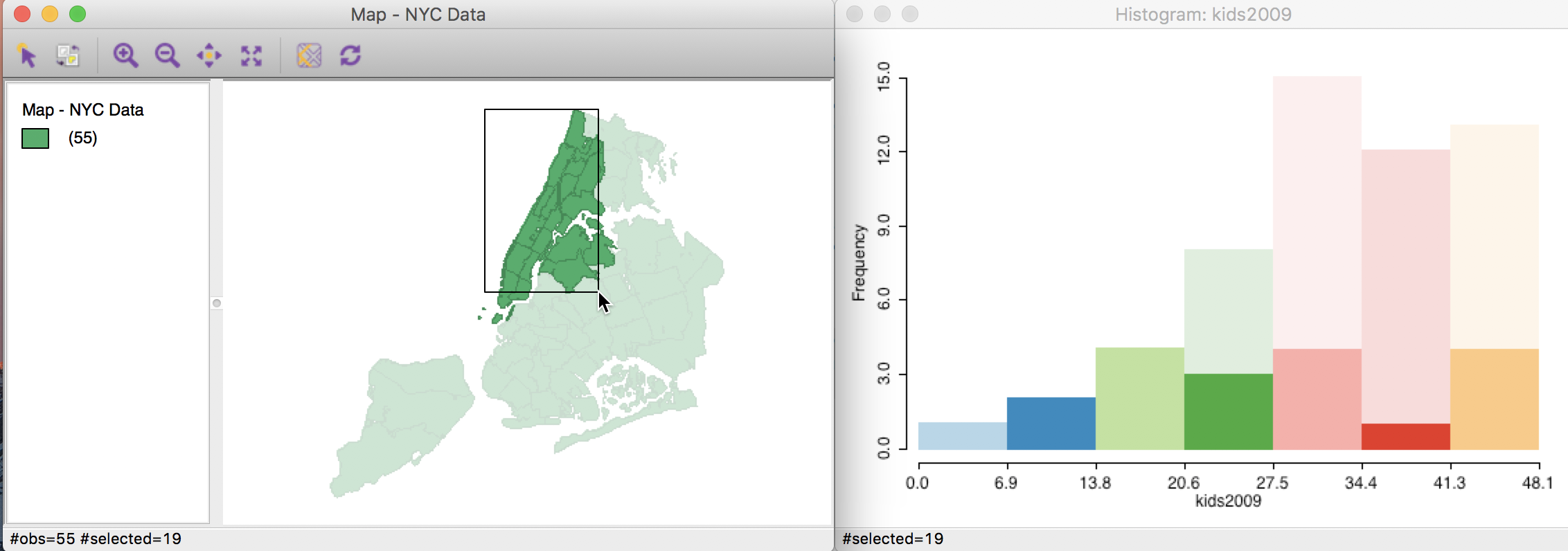Figure 14: Linking a map and a histogram

As we have seen before, it is also possible to save the selection in the form of a 0-1 indicator variable with the Save Selection option.

The technique of linking, and its dynamic counterpart of brushing (more later) is central to the data exploration philosophy that is behind GeoDa (for a more elaborate exposition of the philosophy behind GeoDa, see Anselin, Syabri, and Kho 2006).

#### Saving a graph as an image

A useful option associated with any graph in GeoDa is the possibility to save the graph as an image, in either png (the default) or bmp format. This process is started by selecting Save Image As from the options menu (right click on the graph and select the item as shown in Figure 15).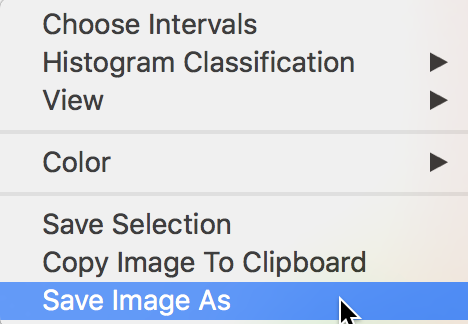Figure 15: Save image as option

The resulting dialog provides a way to specify a file name and a location where to save the file. The default file name in our example is NYC DataHistogramFrame.png. For the histogram without descriptive statistics, with 7 categories and selections highlighted, the corresponding image is as shown in Figure 16. This makes it easy to incorporate the graphs into a document.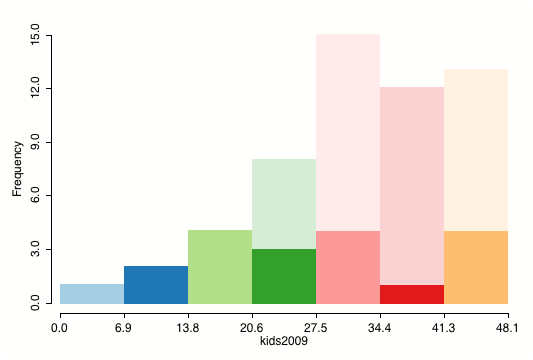Figure 16: Histogram as png image

### Box plot

A box plot is an alternative visualization of the distribution of a single variable. It is invoked as Explore > Box Plot, or by selecting the Box Plot icon in the toolbar, shown in Figure 17.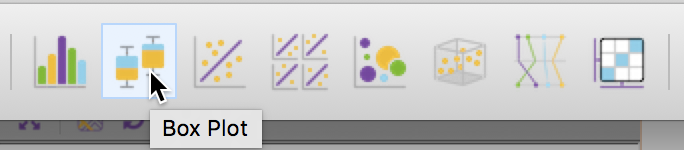Figure 17: Box plot toolbar icon

Identical to the approach followed for the histogram, next appears a Variable Settings dialog to select the variable. In GeoDa, the default is that the variable from any previous analysis is already selected. In our example, we continue with kids2009 (see Figure 6). This brings up the box plot graph shown in Figure 18 (make sure to turn off any previous selection of observations).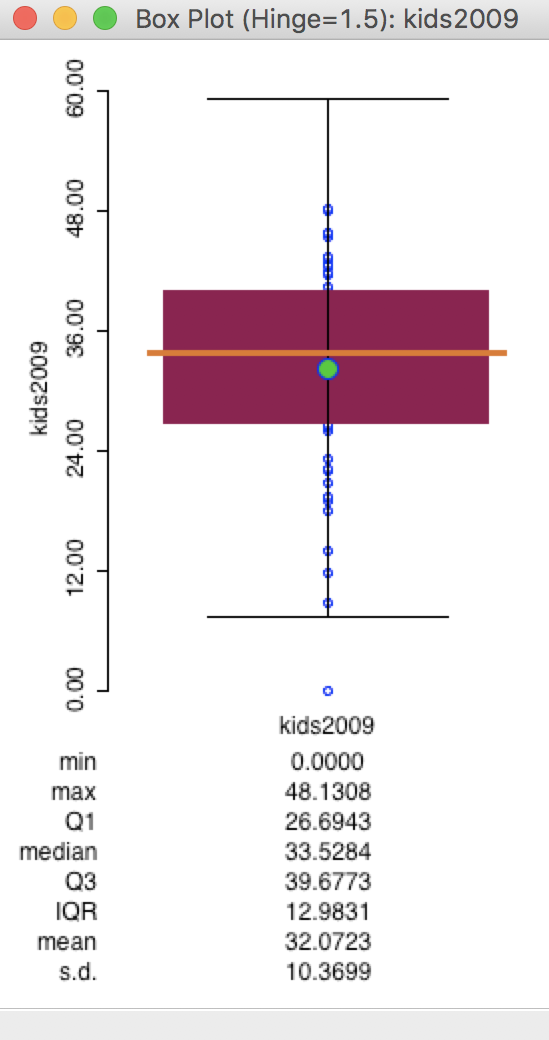Figure 18: Default box plot

The box plot focuses on the quantiles of the distribution. The data points are sorted from small to large. The median (50 percent point) is represented by the horizontal orange bar in the middle of the distribution. The brown rectangle goes from the first quartile (25th percentile) to the third quartile (75th percentile). The difference between the values that correspond to the third (39.6773) and the first quartile (26.6943) is referred to as the inter-quartile range (IQR). The inter-quartile range is a measure of the spread of the distribution, a non-parametric counterpart to the standard deviation. In our example, the IQR is roughly 13 (39.6773 - 26.6943 = 12.9831).

The horizontal lines drawn at the top and bottom of the graph are the so-called fences or hinges. They correspond to the values of the first quartile less 1.5xIQR (i.e., roughly 26.7 - 1.5x13 = 7.2), and the third quartile plus 1.5xIQR (i.e., roughly 39.7 + 1.5x13 = 59.2). Observations that fall outside the fences are considered to be outliers.3

In our example in Figure 18, we have a single lower outlier, but no upper outliers. Note that the one lower outlier is the observation that corresponds with a value of 0 (the minimum), which we earlier had flagged as potentially suspicious. The outlier detection would seem to confirm this. Checking for strange values that may possibly be coding errors or suggest other measurement problems is one of the very useful applications of a box plot.

The default in GeoDa is to list the summary statistics at the bottom of the box plot. As was the case for the histogram, the statistics include the minimum, maximum, mean, median and standard deviation. In addition, the values for the first and third quartile and the resulting IQR are given as well. The listing of descriptive statistics can be turned off by unchecking View > Display Statistics (i.e., the default is the reverse of what held for the histogram, where the statistics had to be invoked explicitly).

The typical multiplier for the IQR to determine outliers is 1.5 (roughly equivalent to the practice of using two standard deviations in a parametric setting). However, a value of 3.0 is fairly common as well, which considers only truly extreme observations as outliers. The multiplier to determine the fence can be changed with the Hinge > 3.0 option (right click in the plot to select the options menu, and then choose the hinge value, as in Figure 19).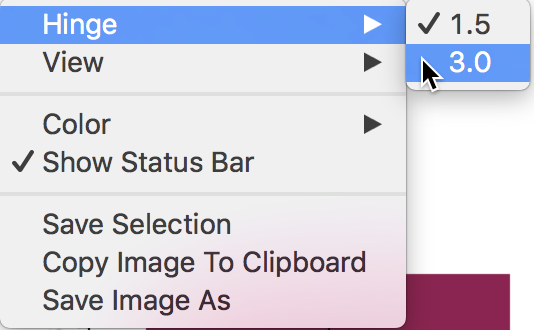Figure 19: Change the box plot hinge

The resulting box plot (with the statistics display turned off in Figure 20) no longer characterizes the lowest value as an outlier.Figure 20: Box plot with hinge = 3.0

Several other options for the box plot are the same as for the histogram, such as saving the selection, copying the image to the clipboard, and saving the graph as an image file.

Also, as is the case for any graph in GeoDa, linking is implemented. For example, selecting the lower outlier in the box plot will highlight the corresponding observation in the themeless map of sub-boroughs, as shown in Figure 21. The reverse process, consisting of first selecting in the map, works as well.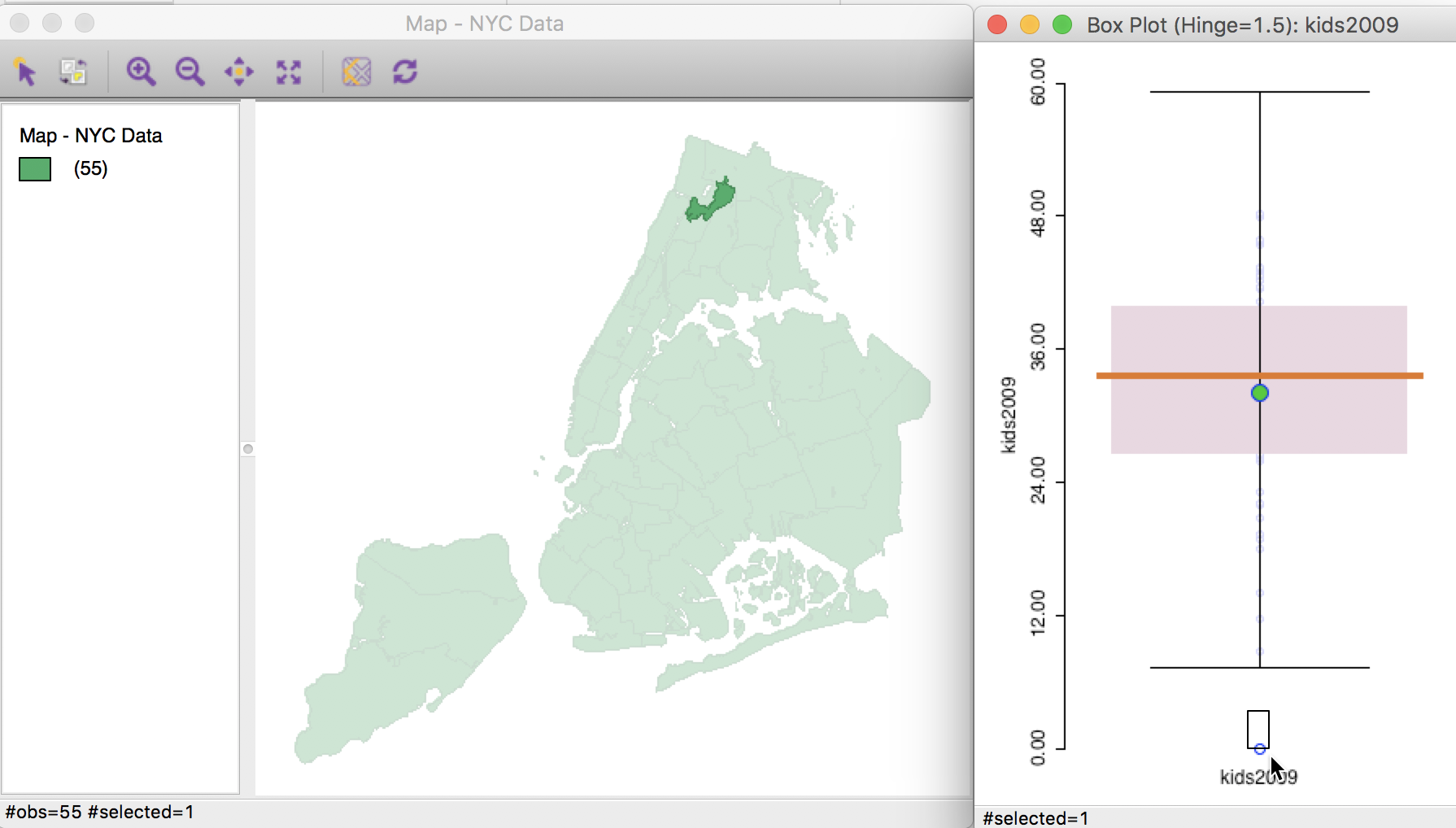Figure 21: Showing the outlier on a linked map

The main purpose of the box plot in an exploratory strategy is to identify outlier observations. Later, we will see how to assess whether such outliers also coincide in space.

## Bivariate Analysis: The Scatter Plot

### Creating a Scatter Plot

The standard tool to assess a linear relationship between two variables is the scatter plot, a diagram with two axes, each corresponding to one of the variables. The observation (x, y) pairs are plotted as points in the diagram.

We create a scatter plot by clicking on its toolbar icon, or by selecting Explore > Scatter Plot from the menu. The Scatter Plot icon is the third in the EDA group on the toolbar, shown in Figure 22.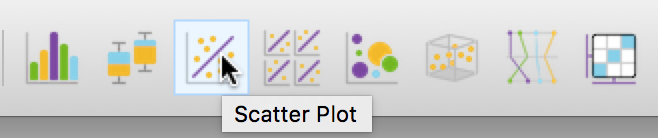Figure 22: Scatter Plot toolbar icon

This brings up the Scatter Plot Variables dialog where the variables for the X and Y axes need to be selected. In our example, illustrated in Figure 23, we will choose the percentage of households with kids under age 18 in 2000 (kids2000) as the X-variable, and the percentage of households receiving public assistance (pubast00) as the Y-variable.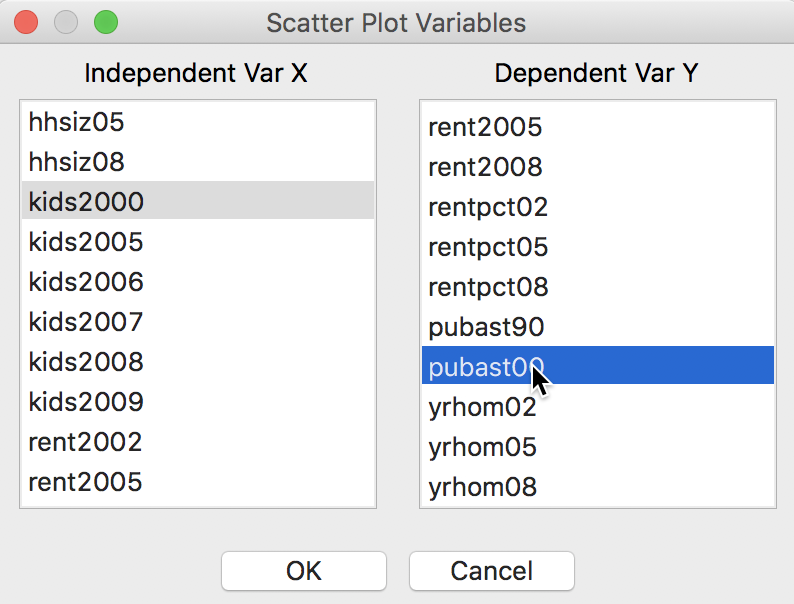Figure 23: Scatter Plot variable selection

Clicking OK brings up the scatter plot.

The default view of the scatter plot is to use the variables in their original scales (i.e., not standardized), show the axes through zero (as dashed lines), and fit a linear smoother (i.e., a least squares regression fit). In our example in Figure 24, there is only a horizontal axis, since all the x values are larger than zero.

In addition, at the bottom of the graph, several summary statistics are listed for the regression line. This includes the R2 of the fit, and the estimate, standard error, t-statistic and p-value for both the intercept and the slope coefficient. This default view is illustrated in Figure 24.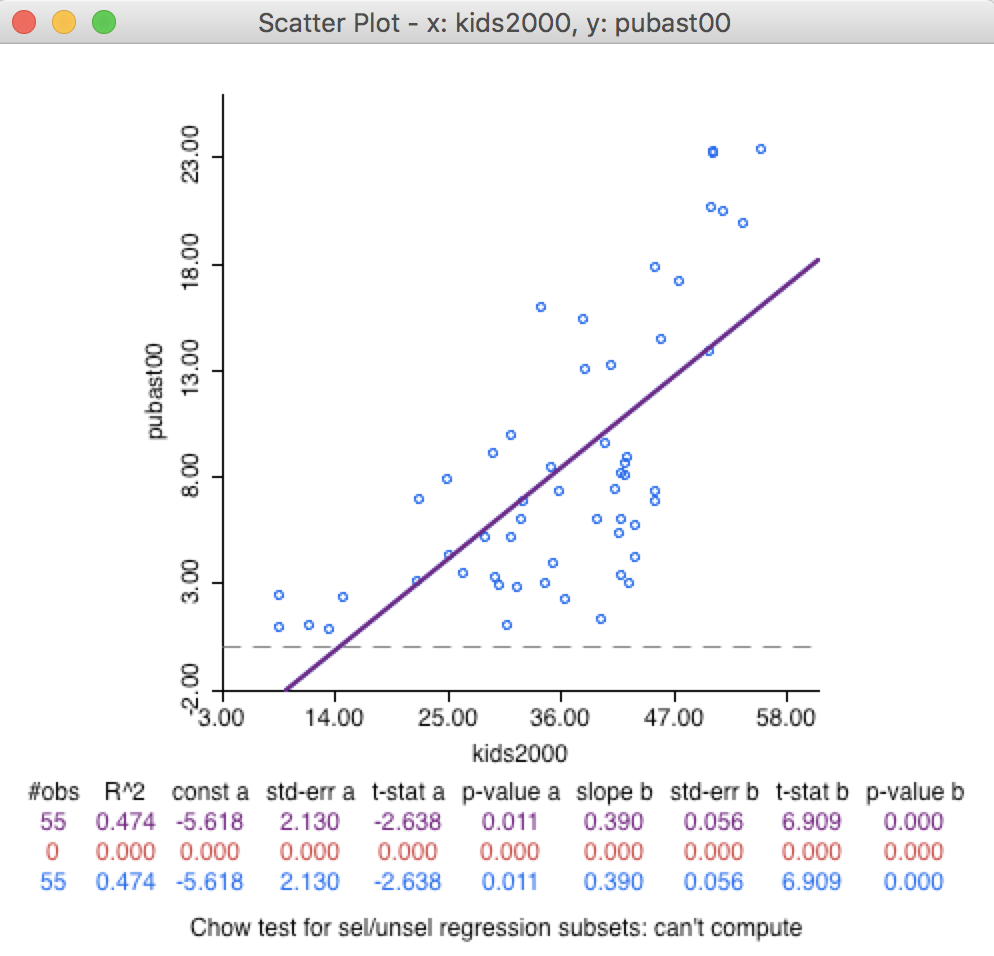Figure 24: Default Scatter Plot

In the current setup, no observations are selected, so that the second line in the statistical summary in Figure 24 (all red zeros) has no values. This line pertains to the selected observations. The blue line at the bottom relates to the unselected observation. The sum of the number of observations in each of the two subsets always equals the total number of observations, listed on the top line. The three lines are listed because of the default View setting of Regimes Regression, even though there is currently no active selection (see also below).

#### Scatter Plot options

The scatter plot has several interesting options, listed in Figure 25. As usual, these are brought up by right clicking in the view or by selecting Options in the menu.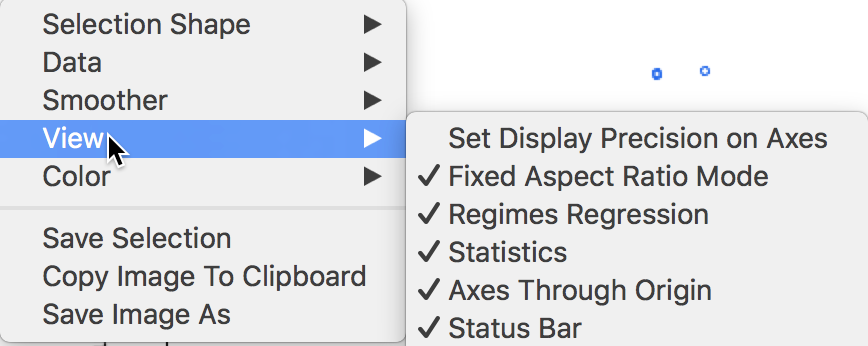Figure 25: Scatter Plot options

Several of the options should by now be familiar, such as the Selection Shape, Color, Save Selection and the two ways to save the image. The Data item provides a choice between the variables on their original scale (the default) and the use of standardized variables. Note that when you use the standardized form, the slope of the linear smoother is also the correlation coefficient between the two variables.

The View option shows the default settings with the Statistics displayed below the graph, the Axes Through Origin shown as dashed lines, and the Status Bar active. Two other default settings are to have a Fixed Aspect Ratio and Regimes Regression active. When some observations are selected, the regimes regression setting will result in the computation of three different linear smoothers. We revisit this when we consider brushing the scatter plot.

The first of the view options is not set by default. It controls the precision by which the values are displayed on the axes. In our scatter plot in Figure 24, this is currently two digits. When checking Set Display Precision on Axes, a dialog pops up. For example, we can turn the precision to 1 digit, as in Figure 26.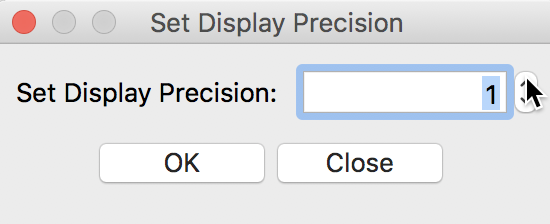Figure 26: Display precision for scatter plot axes

The values displayed on the axes are adjusted in accordance with the new precision setting, shown in Figure 27.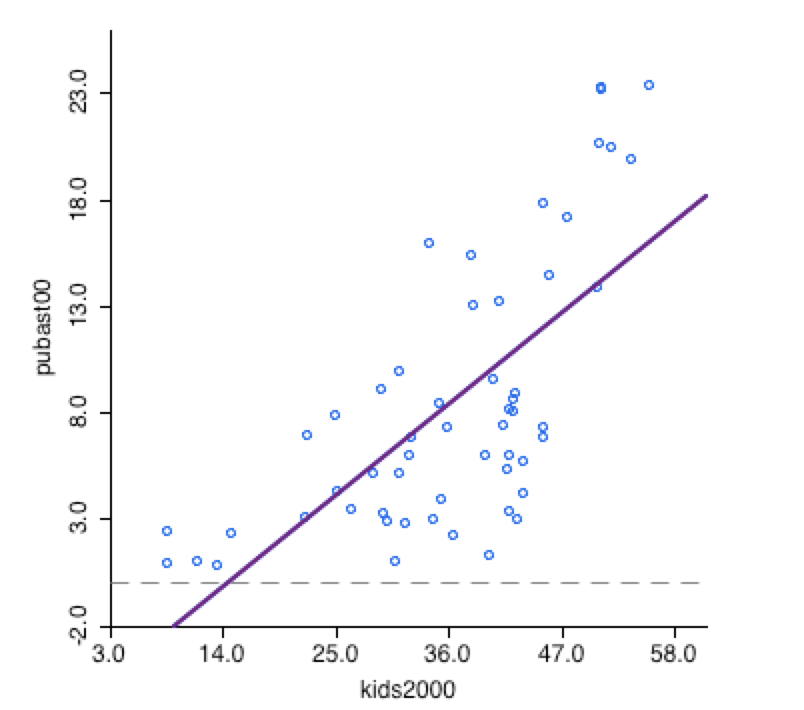Figure 27: Scatter Plot with different precision

#### LOWESS smoother

We turn the precision back to 2 digits and explore a non-linear smoother of the scatter plot. A LOWESS nonlinear local regression fit reveals potential nonlinearities in the bivariate relationship and may suggest the presence of structural breaks (for a good overview of the methodological issues, see, e.g., Cleveland 1979; and Loader 1999, 2004). LOWESS stands for locally weighted scatter plot smoother and is slightly different from LOESS, a local polynomial regression (the two are often confused, but implement different fitting algorithms). In GeoDa, LOWESS is implemented and selected in the Smoother option, as shown in Figure 28.Figure 28: Scatter Plot smoothing options

Selecting the Show LOWESS Smoother option adds the nonlinear fit to the scatter plot. Note that by default the Show Linear Smoother option remains checked, so that this needs to be unchecked to see only the nonlinear fit. The respective graphs that result from each option are illustrated in Figures 29 and 30. In practice, having both options selected facilitates an easy comparison of the two curve fits.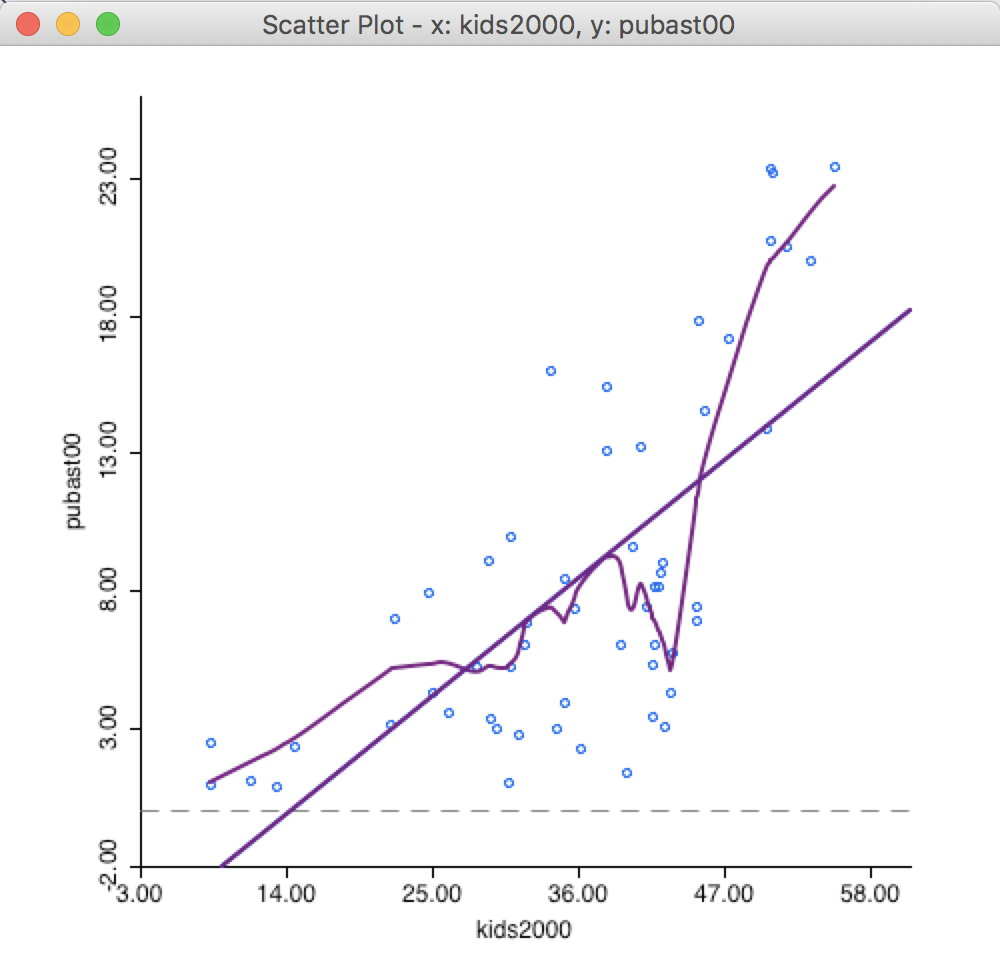Figure 29: Default LOWESS smoother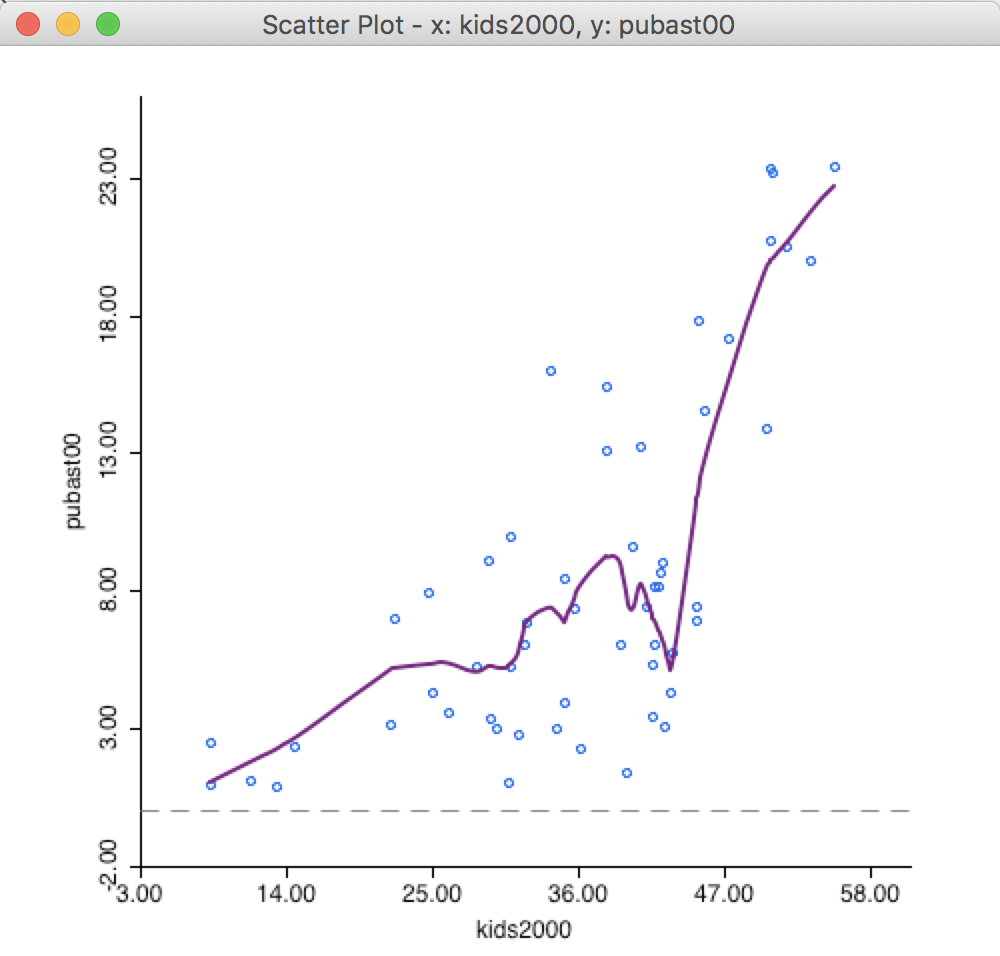Figure 30: LOWESS smoother without linear fit

In our example, there is considerable evidence of a nonlinear relationship between the two variables. An alternative interpretation is to see this as an indication of a structural break, where in one subset of the data the slope is very steep, whereas in another it is fairly flat.

The nonlinear fit is driven by a number of parameters, the most important of which is the bandwidth. The parameters can be changed in the options by selecting Edit LOWESS Parameters in the Smoother option. As shown in Figure 31, a small dialog is brought up in which the Bandwidth (default setting 0.20), Iterations and Delta Factor can be adjusted. The bandwidth determines the smoothness of the curve and is given as a fraction of the total range in X values. In other words, the default bandwidth of 0.20 implies that for each local fit (centered on a value for X), about one fifth of the scatter points are taken into account. The other options are technical and are best left to their default values.4

To obtain Figure 32, we changed the bandwidth to 0.40, which results in a much smoother curve that brings out a possible structural break in the data in a more striking fashion.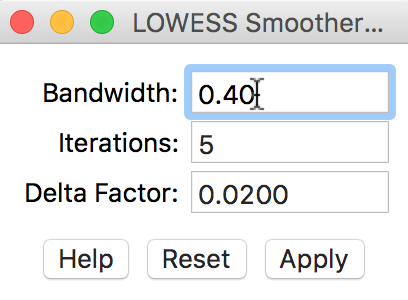Figure 31: LOWESS bandwidth settings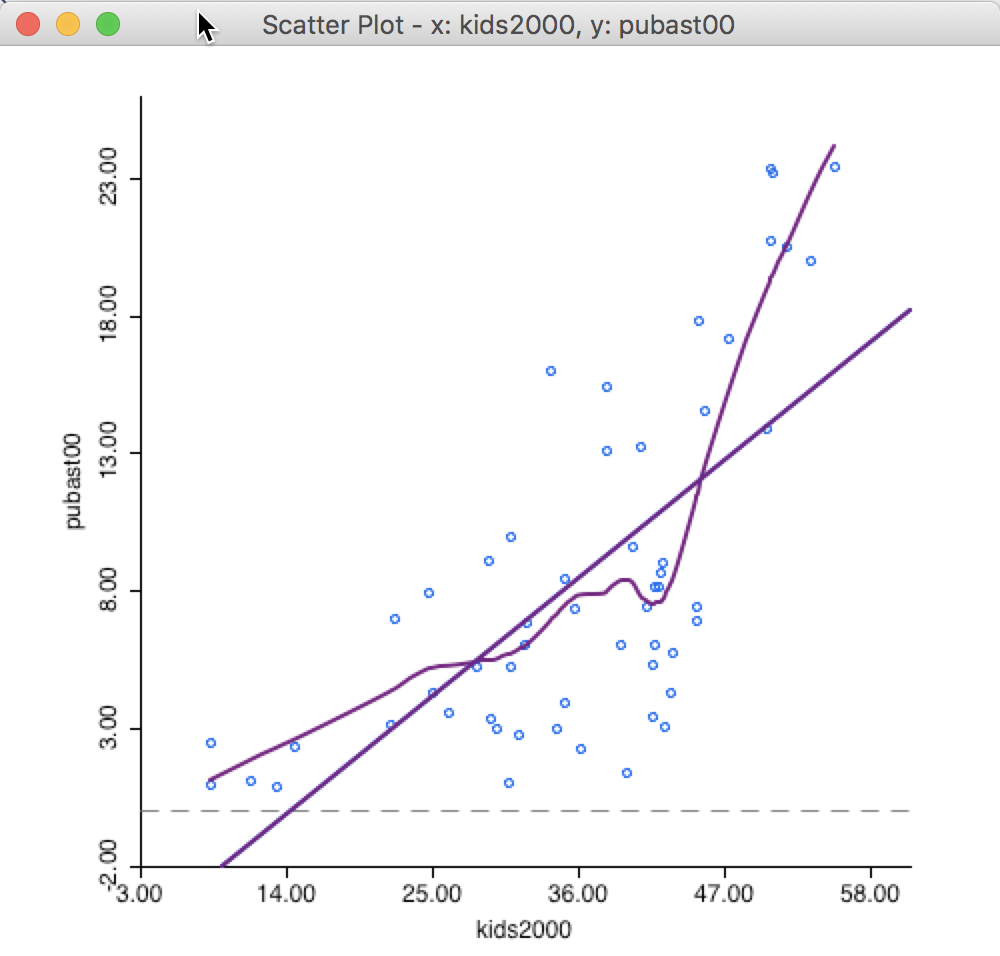Figure 32: LOWESS smoother bandwidth 0.40

The plot seems to suggest that the linear fit is really a compromise between two slopes. There is a steep slope for observations with a value for households with children above 40 percent, suggesting a major increase in public assistance with every increase in the percentage children. With values for kids2000 below 40, the slope is much gentler and even flat in small subsets of the data.

The opposite effect is obtained when the bandwidth is made smaller. For example, with a value of 0.10, the resulting curve in Figure 33 is much more jagged and less informative.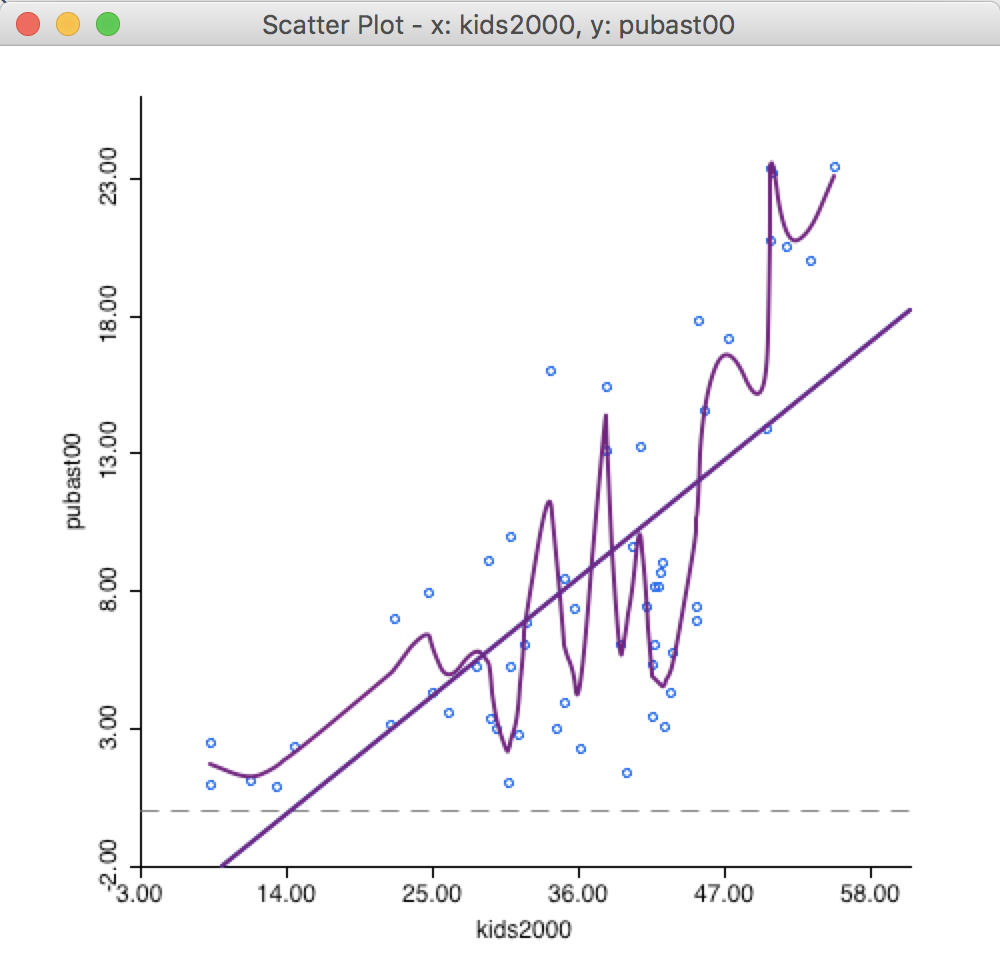Figure 33: LOWESS smoother bandwidth 0.10

The literature contains many discussions of the notion of an optimal bandwidth, but in practice a trial and error approach is often more effective. In any case, a value for the bandwidth that follows one of these rules of thumb can be entered in the dialog. Currently, GeoDa does not compute optimal bandwidth values.

Finally, while one might expect the LOWESS fit and a linear fit to coincide with a bandwidth of 1.0, this is not the case. The LOWESS fit will be near-linear, but slightly different from a standard least squares result due to the locally weighted nature of the algorithm.

### Brushing the Scatter Plot – Spatial Heterogeneity

Linking and brushing are powerful techniques to assess structural breaks in the data, such as evidence of spatial heterogeneity. We have already seen how a selection in any of the views results in the same observation to immediately be selected in all other views through linking. Brushing is a dynamic extension of this process. This is the most insightful when applied to the combination of a map and a scatter plot, but it equally applies to all the other views (for some early exposition and discussion of these ideas pertaining to so-called dynamic graphics, see, e.g., the classic references of Stuetzle 1987; Becker and Cleveland 1987; Becker, Cleveland, and Wilks 1987; Monmonier 1989; as well as in the outline of legacy GeoDa functionality in Anselin, Syabri, and Kho 2006).

The brushing process is initiated by setting up a selection shape in one of the views. The default is a rectangular shape, but we have seen earlier how that can be changed to a circle or a line. In our example, we keep the default. Click anywhere in the scatter plot and draw the pointer into a rectangular shape, as shown below. Note how the pointer is attached to a corner of the rectangle. At this point, the shape can be moved around in the view, dynamically changing the selection.

In our example in Figure 34, we have selected 22 observations. The purple line represents the original linear fit, the red line is the fit for the 22 selected observations, and the blue line is the fit for the other 33 observations. Below the three lines with the slope coefficients and fit statistics, the results are listed of a Chow test on structural stability are listed (Chow 1960). Clearly, in contrast to the overall purple and the blue line, there is no relationship at all for the selected observations in question, as evidenced by the horizontal red line. The Chow test confirms this by strongly rejecting (p < 0.0005) the null hypothesis of equal coefficients (between the slopes of the blue and the red lines).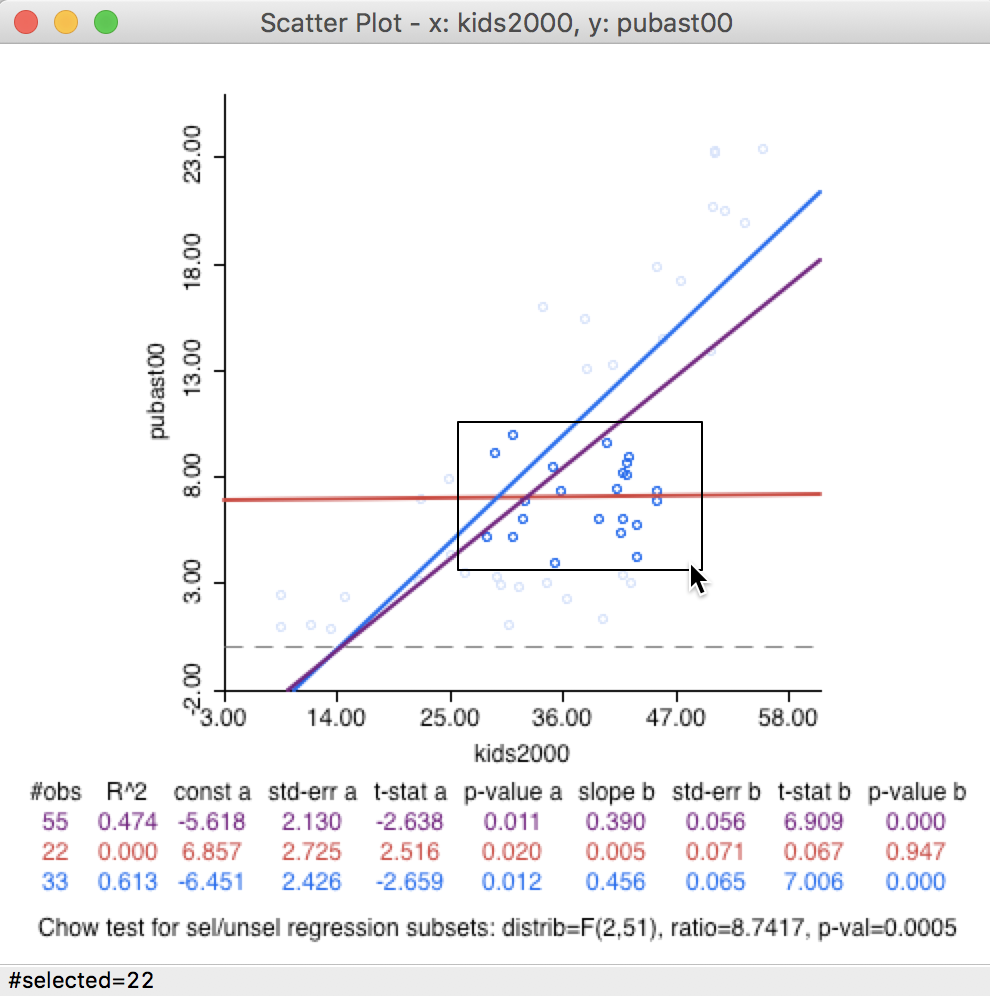Figure 34: Brushing the scatter plot – 1

Because of the linking, the 22 selected observations are also highlighted in all the other views, such as the green themeless map shown in Figure 35. In an actual application, this map can be for a third variable, allowing us to investigate potential interaction effects.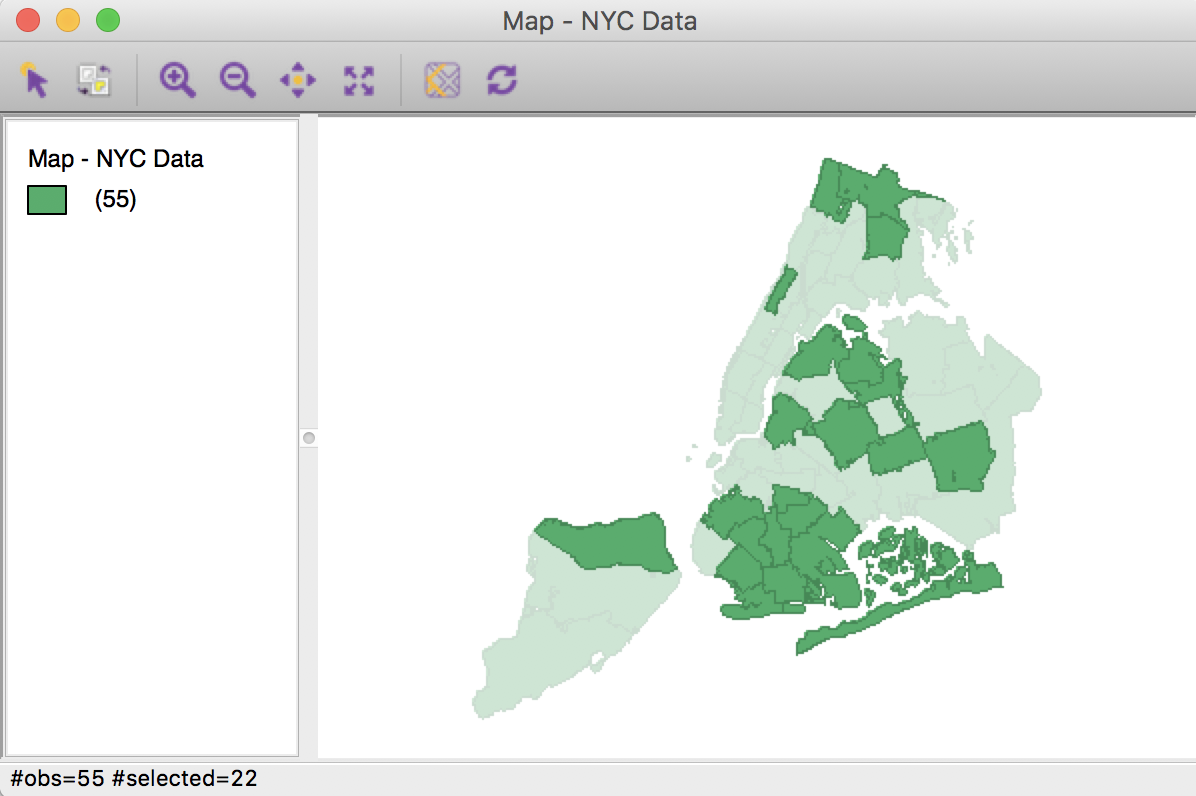Figure 35: Linked map selection – 1

With the Regimes Regression option turned on, the three linear fits change instantaneously as different observations are selected. Of course, the fits themselves are only meaningful when sufficient observations are part of the selection. For example, we can move the selection rectangle up and to the right, as in Figure 36, which yields a new selection of 10 observations, with associated regression lines. This time, there is insufficient evidence to reject the null hypothesis (Chow test with p = 0.975).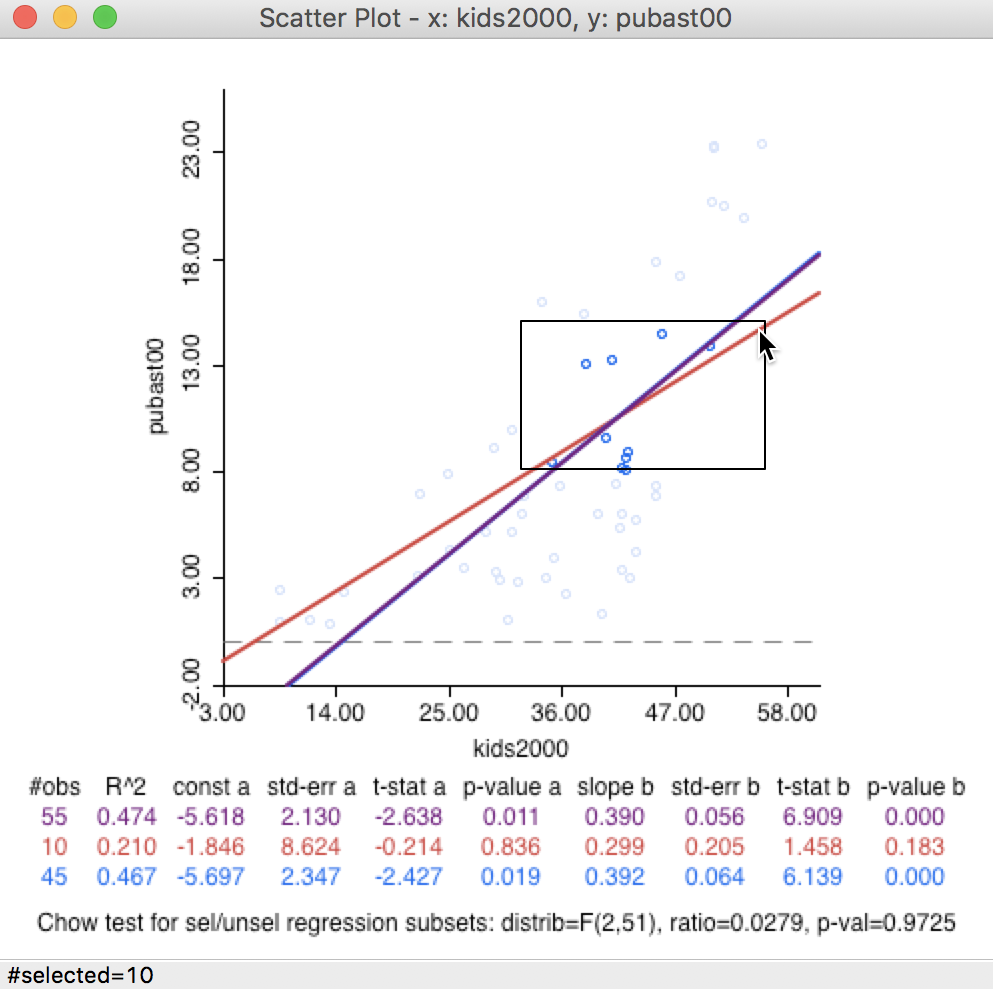Figure 36: Brushing the scatter plot – 2

Again, the matching locations are shown in the map in Figure 37. As the selection rectangle moves in the scatter plot, the highlighted sub-boroughs in the map change as well.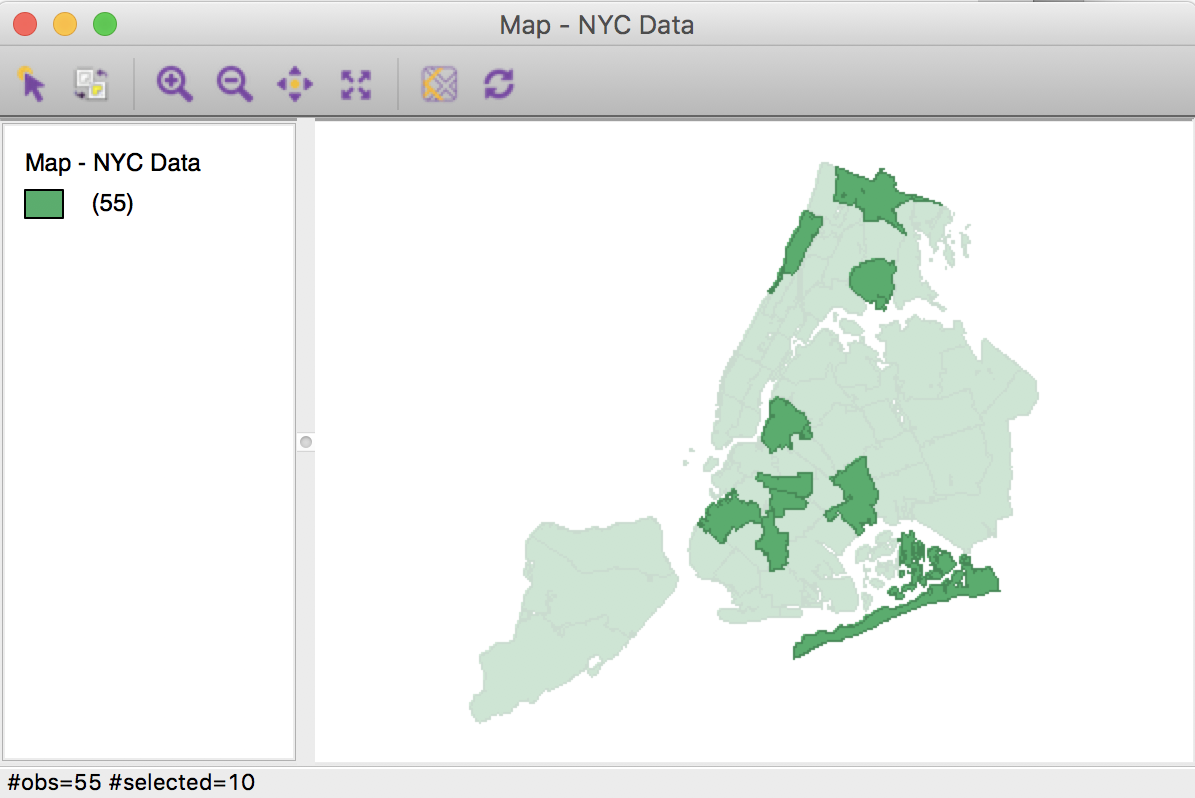Figure 37: Linked map selection – 2

The process can also be reversed and started in a view other than the scatter plot. For example, we can brush the map (in our example in Figure 38, 10 observations are selected), and assess how the linear fits are affected in the scatter plot.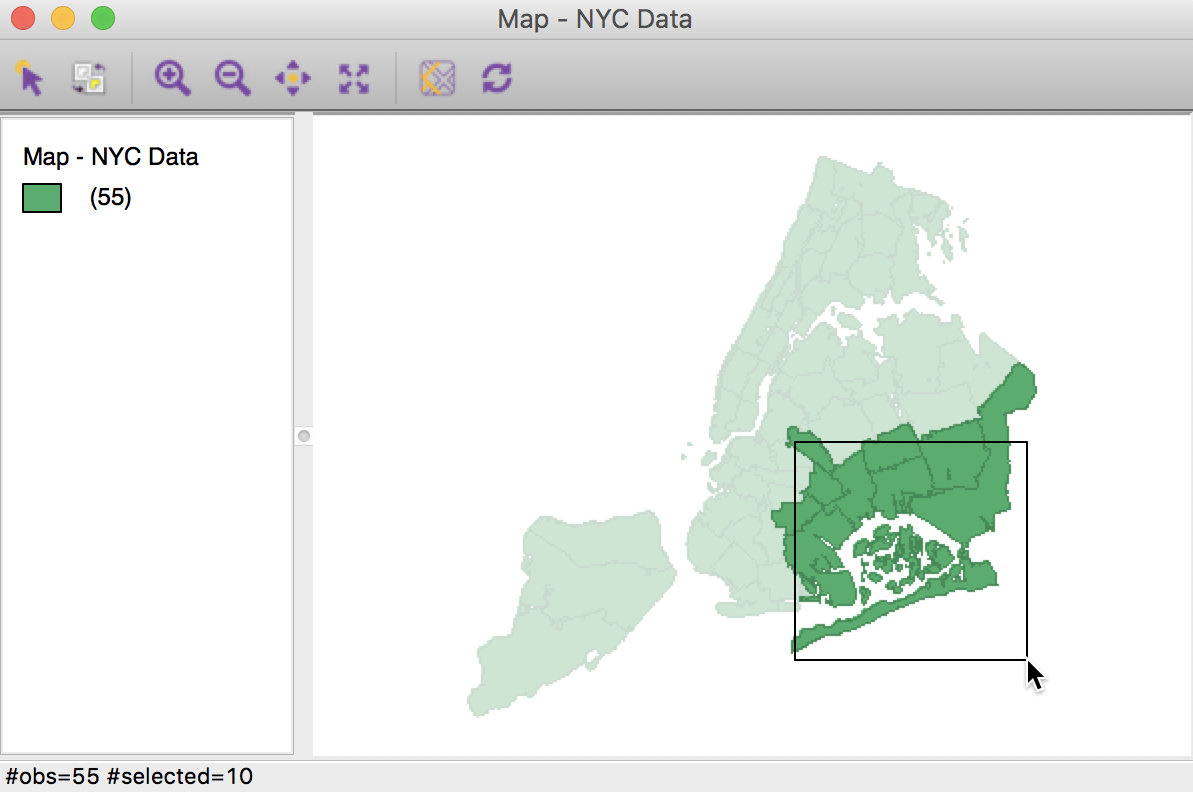Figure 38: Brushing the map – 1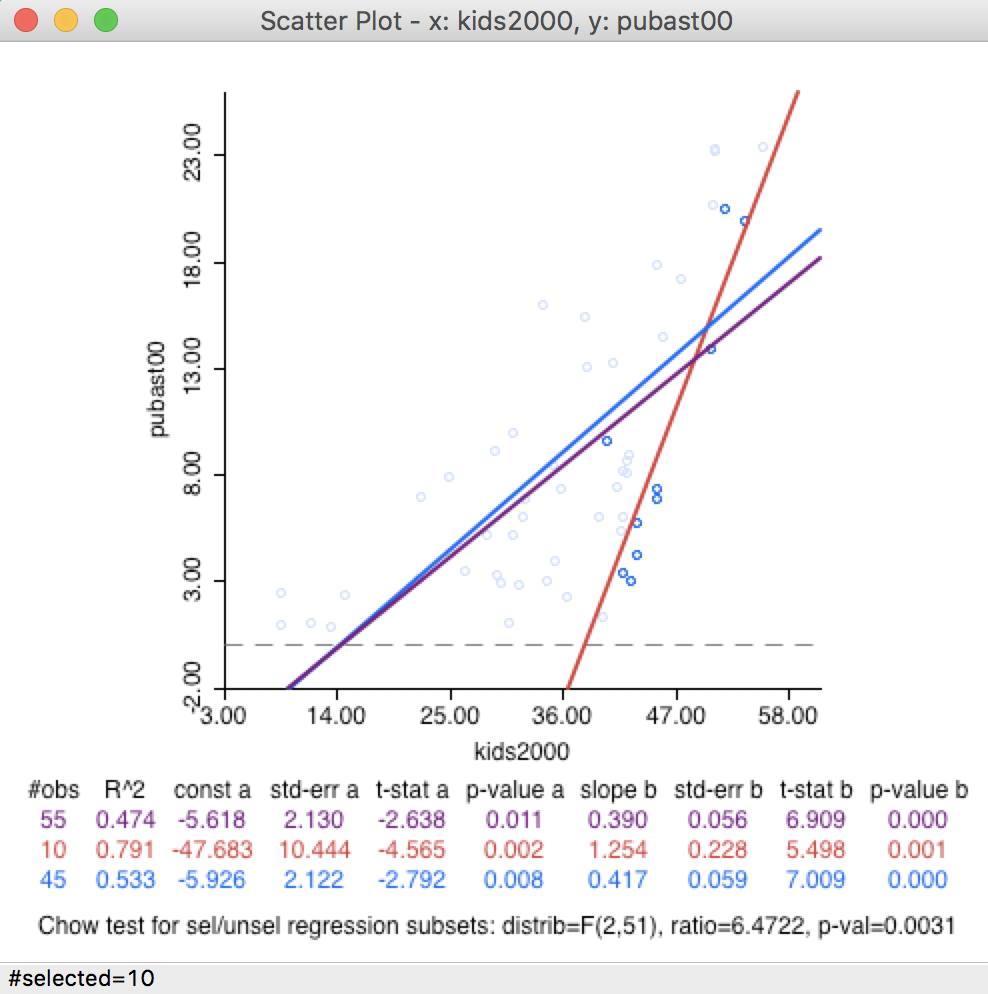Figure 39: Linked scatter plot selection – 1

In Figure 39, the map selection results in a rejection of the null hypothesis of constant slopes with p < 0.0031. In other words, the slope in the region we selected in the map is significantly different from the slope in the rest of the map, suggesting spatial heterogeneity.

As we brush across the map, we can assess the degree to which the linear relationship is stable. Any systematically changing slopes between clearly defined sub-regions of the observations would suggest the presence of spatial heterogeneity. For example, moving the selection rectangle north, as in Figure 40, makes the evidence for structural change somewhat weaker (as evidenced by the Chow test with p < 0.01 shown in Figure 41).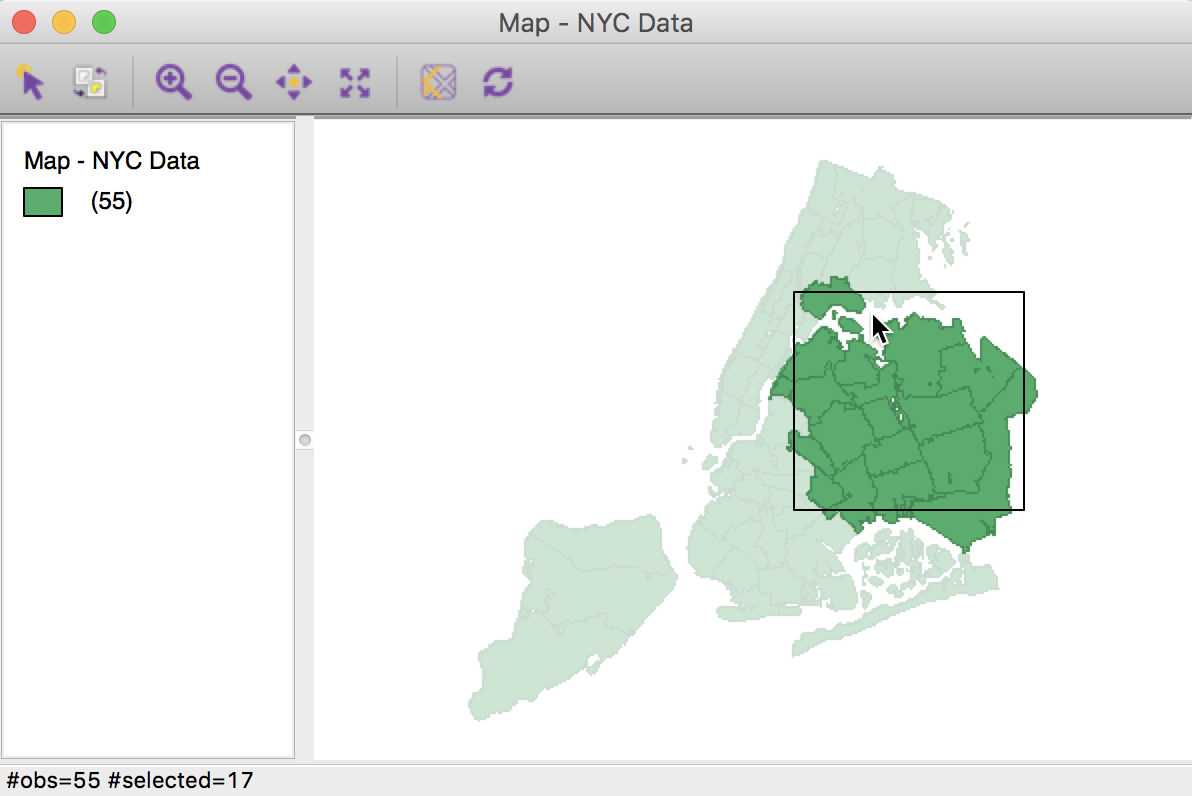Figure 40: Brushing the map – 2Figure 41: Linked scatter plot selection – 2

As we identify subregions in the data with a different slope (structure) from the rest, we can assess this more formally through regression analysis (e.g., analysis of variance). This is facilitated by Saving the selection in the form of an indicator variable (with 1 for the selected observations). This new variable can then be incorporated in a regression specification.

1. University of Chicago, Center for Spatial Data Science – anselin@uchicago.edu

2. In the GeoDa Preference Setup, under System, the transparency of the unhighlighted objects in a selection operation can be adjusted. The default is 0.80, which means only about 20% of the regular color is shown.

3. Note that the fences are drawn even when they fall outside the actual range of the observations. This will be the case whenever the value of the third quartile + 1.5xIQR is larger than the maximum, (as in in Figure 18), or when the value of the first quartile - 1.5xIQR is smaller than the minimum.

4. The LOWESS algorithm is complex and uses a weighted local polynomial fit. The Iterations setting determines how many times the fit is adjusted by refining the weights. A smaller value for this option will speed up computation, but result in a less robust fit. The Delta Factor drops points from the calculation of the local fit if they are too close (within Delta) to speed up the computations. Technical details are covered in Cleveland (1979).